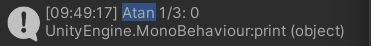# How do I calculate an atan of a value less than 1?

Unsing Unity 2021.3.9f1

My code:

``````print("Atan 1/3: "+MathF.Atan(1/3));
``````

My Result:Same thing happens for 1/2.

Whats going on here and how do I get correct results?

BTW: No change in result if I use Mathf or MathF, neither can do it.
Restarting Unity, which has fixed similier miscalculation errors in the past did not do the trick this time.

Thanks

The problem isn’t the Atan but that 1/3 is zero. That’s because 1 and 3 are both integers so the division operator performs integer division (rounding towards zero). You can mark a number literal as a float (32-bit floating point number) with the suffix f:

`Mathf.Atan(1f / 3f)`